Multiplication of Monomials by Binomials and Trinomials

Chapter 8 Class 8 Algebraic Expressions and Identities
Concept wise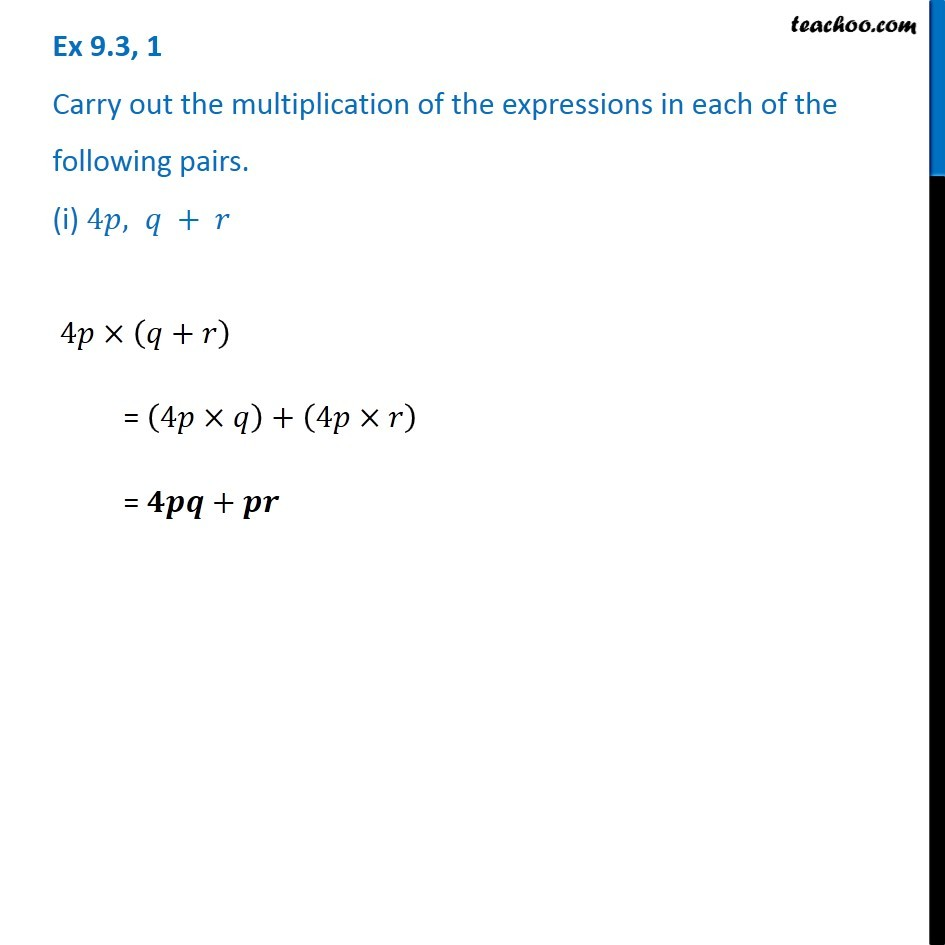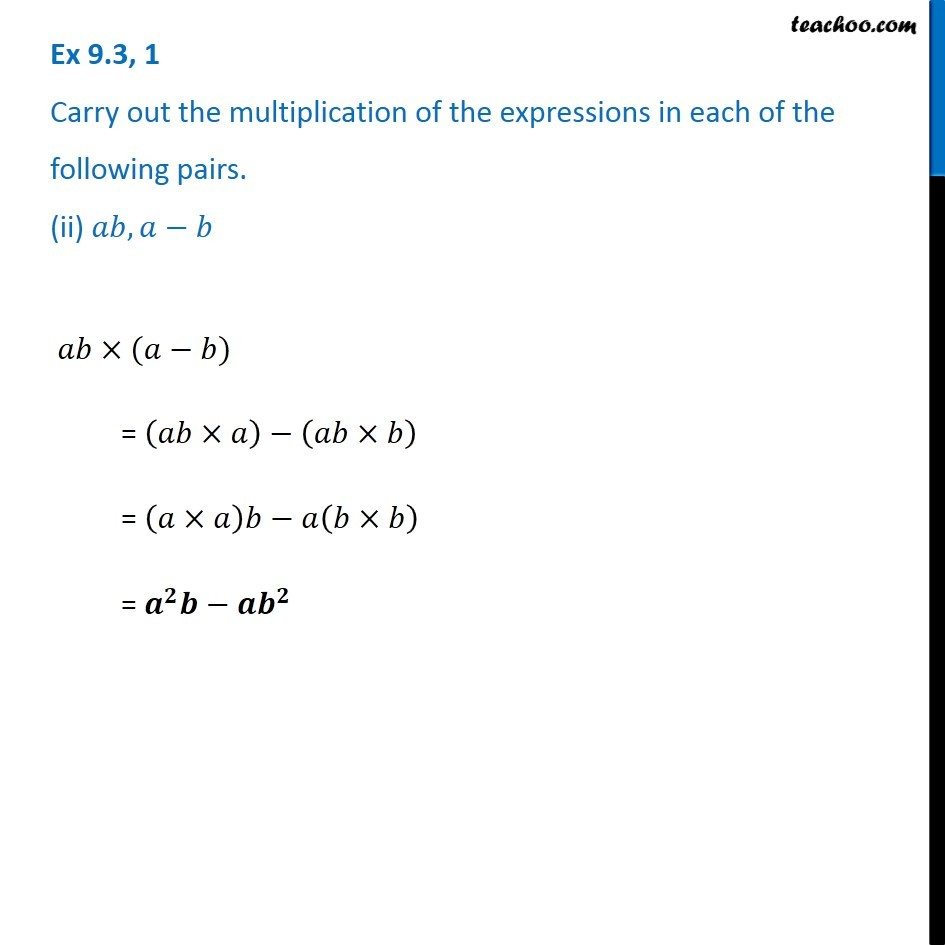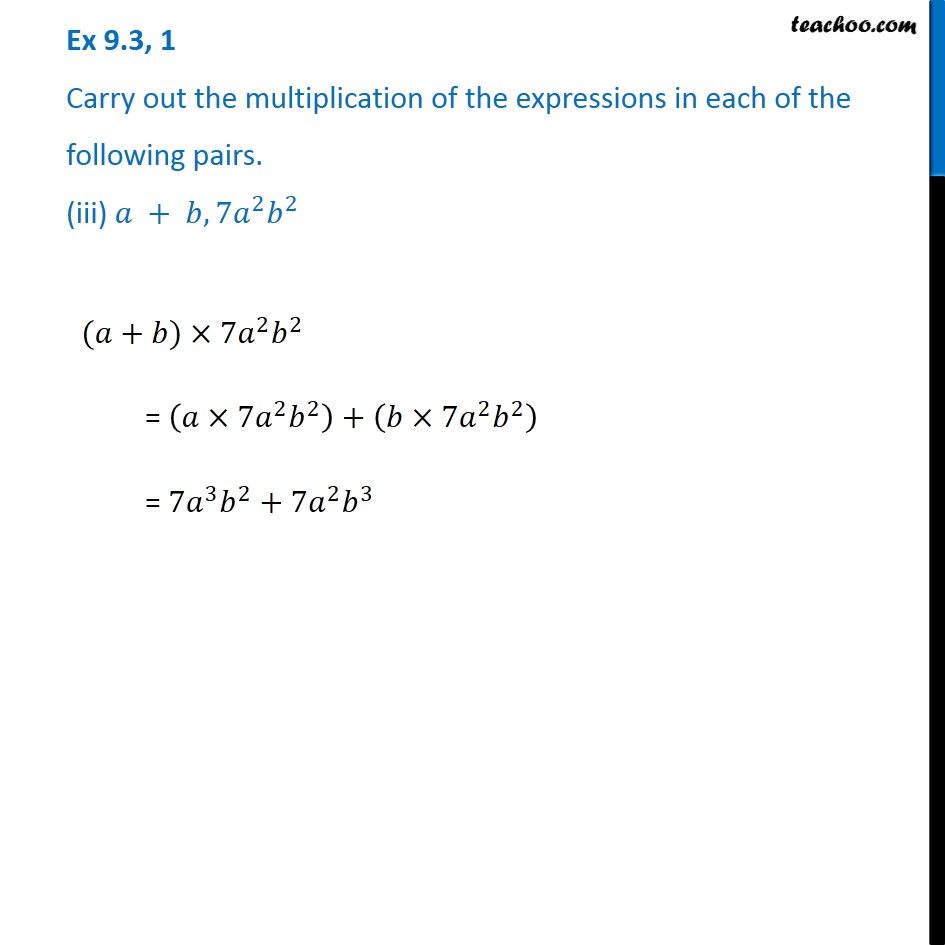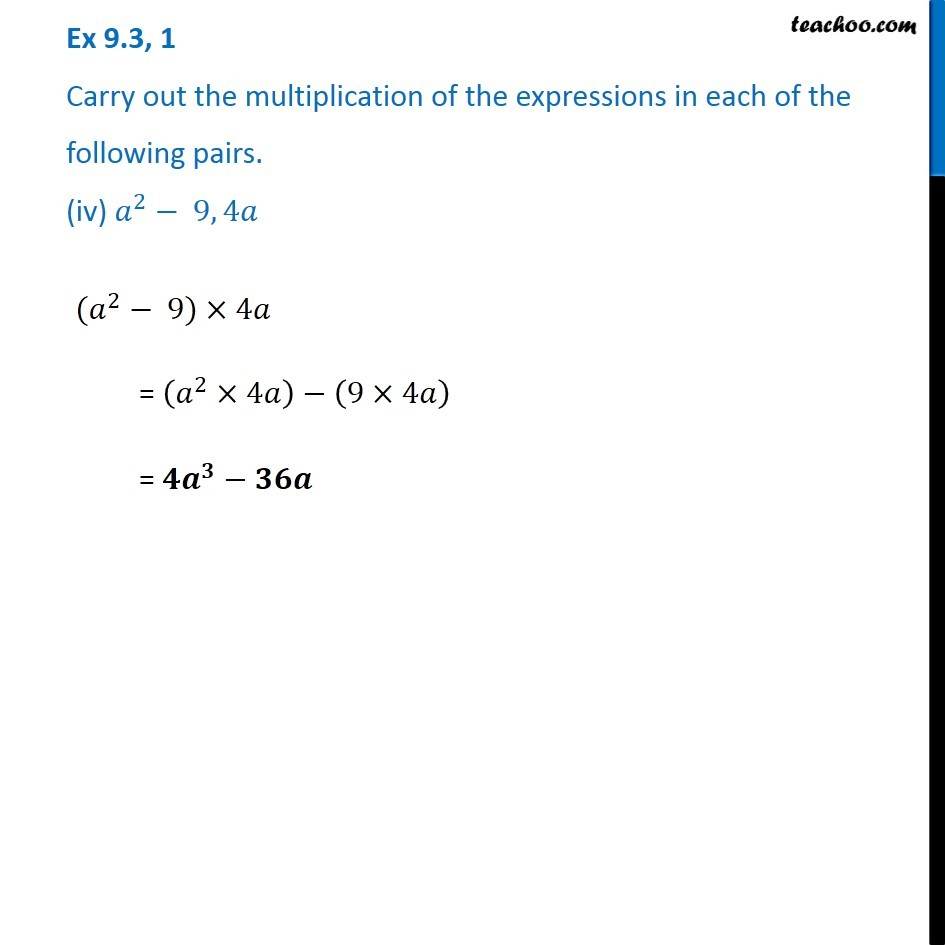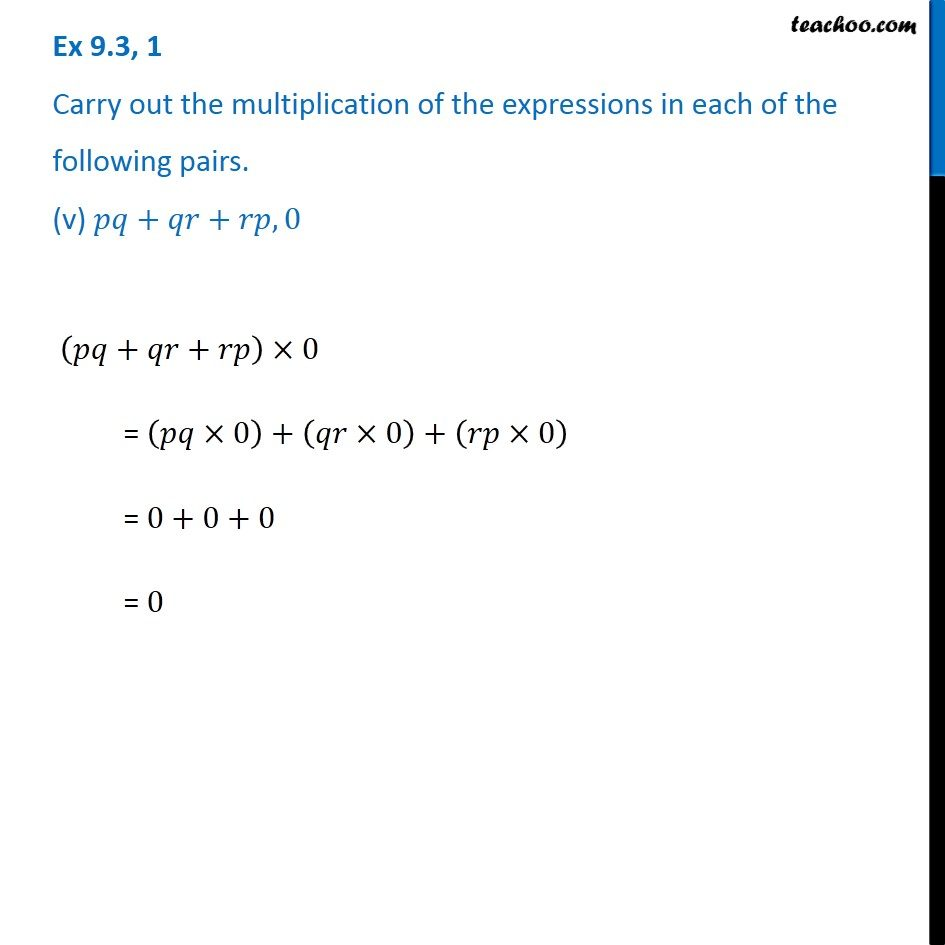Learn in your speed, with individual attention - Teachoo Maths 1-on-1 Class

### Transcript

Ex 8.3, 1 Carry out the multiplication of the expressions in each of the following pairs. (i) 4𝑝, 𝑞 + 𝑟 4𝑝×(𝑞+𝑟) = (4𝑝×𝑞)+(4𝑝×𝑟) = 𝟒𝒑𝒒+𝒑𝒓 Ex 8.3, 1 Carry out the multiplication of the expressions in each of the following pairs. (ii) 𝑎𝑏, 𝑎−𝑏 𝑎𝑏×(𝑎−𝑏) = (𝑎𝑏×𝑎)−(𝑎𝑏×𝑏) = (𝑎×𝑎)𝑏−𝑎(𝑏×𝑏) = 𝒂^𝟐 𝒃−𝒂𝒃^𝟐 Ex 8.3, 1 Carry out the multiplication of the expressions in each of the following pairs. (iii) 𝑎 + 𝑏, 7𝑎^2 𝑏^2 (𝑎+𝑏)×7𝑎^2 𝑏^2 = (𝑎×7𝑎^2 𝑏^2 )+(𝑏×7𝑎^2 𝑏^2 ) = 7𝑎^3 𝑏^2+7𝑎^2 𝑏^3 Ex 8.3, 1 Carry out the multiplication of the expressions in each of the following pairs. (iv) 𝑎^2− 9, 4𝑎 (𝑎^2− 9)×4𝑎 = (𝑎^2×4𝑎)−(9×4𝑎) = 𝟒𝒂^𝟑−𝟑𝟔𝒂 Ex 8.3, 1 Carry out the multiplication of the expressions in each of the following pairs. (v) 𝑝𝑞+𝑞𝑟+𝑟𝑝, 0 (𝑝𝑞+𝑞𝑟+𝑟𝑝)×0 = (𝑝𝑞×0)+(𝑞𝑟×0)+(𝑟𝑝×0) = 0+0+0 = 0